Tortoise and Hares Amazing Race - For Creative Minds Quiz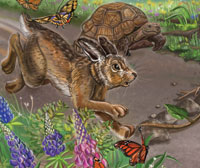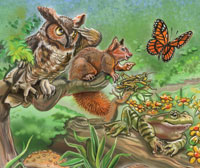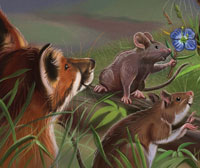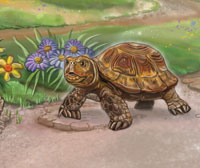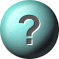1. A mile is a unit of measurement that can measure:     length     time     volume2. Which is the correct order, from smallest to largest     foot, inch, mile, yard     inch, foot, yard, mile     mile, foot, inch, yard3. How many feet are in a mile?     5280     8250     25084. What measurement tool is usually one foot long and marked in inches?     ruler     tape measure     yardstick5. What measurement tool is three feet long?     ruler     tape measure     yardstick6. Which of the following distances is the longest?     50 feet     25 yards     1 mile7. Which of the following distances is the shortest?     60 feet     21 inches     40 yards8. Which of the following symbols means "less than"?     <     >     =9. Which of the following symbols means "greater than"?     <     >     =10. Which of the following symbols means "equal to"?     <     >     =